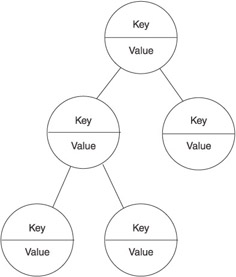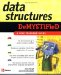# Why Use a Binary Tree?

Programmers use a binary tree to quickly look up data stored at each node on the binary tree. Let s say that you need to find a student ID in a list of a million student IDs. What is the maximum number of comparisons that you ll need to make before finding the student ID?

You could make a maximum of a million comparisons if you sequentially searched the list of a million student IDs. More than a million comparisons are necessary if you randomly selected student IDs from the list and then replaced those that didn t match back into the list.

However, you d need to make a maximum of only 20 comparisons if student IDs were stored in a binary tree. This is because of the way data is organized in a binary tree. Data stored on the left node is less than data stored on all the right nodes at any current node.

This might sound a little confusing, but an example will make this concept clear. Suppose you had a list of five student IDs: 101, 102, 103, 104, and 105. These student IDs are stored in a binary tree so that the center student ID is the root node, the student ID that is less than the current node is stored on the left child node, and the student ID that is more than the current node is stored on the right child node, as shown in Figure 10-4.Figure 10-4: The left child node is always less than the parent node, and the right child node is always greater than the parent node.

The same pattern is applied to each child node. Thus, student ID 101 is the left child of the node that contains student ID 102 because student ID 101 is less than student ID 102. Likewise, student ID 105 is the right node of student ID 104 because student ID is greater than student ID 105.

Let s say you want to locate student ID 101 on the binary tree. First, you compare the value of the root node to student ID 101. There s no match. Because student ID 101 is less than student ID 103, your next comparison uses the left child node. This eliminates the need to compare all the nodes to the right of the node that contains student ID 103. You can ignore half the student IDs because you know that student ID 101 isn t the right node or a child of the right node.

After comparing student ID 101 to student ID 102, you notice two things. First, they don t match. Second, student ID 102 is greater than student ID 101. This means you compare the left child node to student ID 101. You ignore the right child node and subsequent child nodes because they are greater than student ID 101. There aren t any right child nodes of student ID 102 in this example. The next comparison results in a match. So, in a large binary tree, each time you do a comparison, you eliminate another half of the remaining nodes from the search. If you had 1 million nodes in the tree, you would divide 1 million by 2 about 20 times to reduce it down to one node (2 ^ 20 is about 1 million). This way, you can find the node you re looking for by doing about 20 comparisons.

Programmers think of every node as the root node and all subsequent nodes as its own subtree . They approach nodes in this way because functions that deal with trees are recursive. A function works on a child node and performs the same functionality as if the child node is the root node of the entire tree. That is, the value of the child node is compared to the value of its left node and right node to determine which branch of the tree to pursue .

### The Key

Each node of a tree contains a key that is associated with a value similar to the relationship between a primary key of a database and a row within a table of the database. A key is a value that is compared to search criteria. If the index and the search criteria match, then the application retrieves data stored in the row that corresponds to the key. The data is referred to as the value of the node, as shown in Figure 10-5.Figure 10-5: Each node has an index and a value; the index uniquely identifies the node and retrieves the value of a node.

Any data type can be used as the key. We use a string as the key in examples in this chapter, but we could have chosen any data type. Unlike the primary key of a database, the key to the tree doesn t have to be in natural order. That is, the key doesn t have to be in alphabetical order or numerical order. In a typical tree implementation, you would define a comparator to tell the tree how to order the nodes. In our case, we ll use the natural ordering sequence of a string so we can keep our focus on the internal workings of the tree.Data Structures Demystified (Demystified)
ISBN: 0072253592
EAN: 2147483647
Year: 2006
Pages: 90

Similar book on Amazon